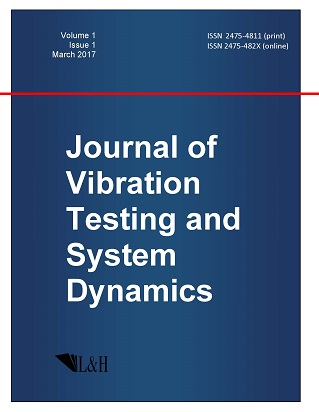ISSN: 2475-4811 (print)
ISSN: 2475-482X (online)
Journal of Vibration Testing and System Dynamics

C. Steve Suh (editor), Pawel Olejnik (editor),

Xianguo Tuo (editor)

Pawel Olejnik (editor)

Lodz University of Technology, Poland

Email: pawel.olejnik@p.lodz.pl

C. Steve Suh (editor)

Texas A&M University, USA

Email: ssuh@tamu.edu

Xiangguo Tuo (editor)

Sichuan University of Science and Engineering, China

Email: tuoxianguo@suse.edu.cn

Mixed Variational Problem for a Generalized Darcy--Forchheimer Model Driven by Hydraulic Fracture

Journal of Vibration Testing and System Dynamics 7(1) (2023) 15--21 | DOI:10.5890/JVTSD.2023.03.003

Victor A. Kovtunenko

Institute for Mathematics and Scientific Computing, University of Graz, NAWI Graz, Heinrichstr.36, 8010 Graz, Austria

Lavrentyev Institute of Hydrodynamics, Siberian Division of the Russian Academy of Sciences, 630090 Novosibirsk, Russia

Abstract

The model of a stationary flow in porous media stemming from hydraulic fracking and accounting for inertial phenomena is considered. The incompressible fluid is modeled by a nonlinear Darcy--Forchheimer (DF) equation under mixed boundary conditions, which are appropriate for a fluid-driven fracture. The classical DF equation is generalized with respect to a growth exponent $m$ and inhomogeneous coefficients. Using mixed variational formulation of the problem for unknown fluid velocity and fluid pressure, the well-posedness theorem is proved for arbitrary $m>1$. The developed Lagrange multiplier formalism is advantageous for optimal shape design of fractures.

References

1.   Cimolina, F. and Discacciati, M. (2013), Navier--Stokes/Forchheimer models for filtration through porous media, Applied Numerical Mathematics, 72, 205-224.
2.   Paranamana, P., Aulisa, E., Ibragimov, A., and Toda, M. (2019), Fracture model reduction and optimization for Forchheimer flows in reservoirs, Journal of Mathematical Physics, 60, 051504.
3.   Skopintsev, A., Dontsov, E., Kovtunenko, P., Baykin, A., and Golovin, S. (2020), The coupling of an enhanced pseudo-3D model for hydraulic fracturing with a proppant transport model, Engineering Fracture Mechanics, 236, 107177.
4.   Itou, H., Hirano, S., Kimura, M., Kovtunenko, V., and Khludnev, A. (2020), Mathematical Analysis of Continuum Mechanics and Industrial Applications III: Proceedings of the International Conference CoMFoS18, vol. 34 of Mathematics for Industry, Springer.
5.   Kovtunenko, V. (2022), Poroelastic medium with a non-penetrating crack driven by hydraulic fracture: variational inequality and its semidiscretization, Journal of Computational and Applied Mathematics, 405, 113953.
6.   Moyo, S., Meleshko, S., and Schulz, E. (2019), Modern Treatment of Symmetries, Differential Equations and Applications (Symmetry 2019), In AIP Conference Proceedings, 2153(1), 010001.
7.   Forchheimer, P. (1930), Hydraulik, Teubner.
8.   Bul{i}\v{c}ek, M., M{a}lek, J., and \v{Z}abensk{y}, J. (2015), A generalization of the Darcy--Forchheimer equation involving an implicit, pressure-dependent relation between the drag force and the velocity, Journal of Mathematical Analysis and Applications, 424, 785-801.
9.   Girault, V. and Wheeler, M. (2008), Numerical discretization of a Darcy--Forchheimer model, Numerische Mathematik, 110, 161-198.
10.   Gutt, R., Kohr, M., Mikhailov, S., and Wendland, W. (2017), On the mixed problem for the semilinear Darcy--Forchheimer--Brinkman PDE system in besov spaces on creased lipschitz domains, Mathematical Methods in the Applied Sciences, 40, 7780-7829.
11.   Khludnev, A. and Kovtunenko, V. (2000), Analysis of Cracks in Solids, vol.~6, WIT-Press.
12.   Ladyzhenskaya, O. (1969), The Mathematical Theory of Viscous Incompressible Flow, Science Publishers.
13.   Cakoni, F. and Kovtunenko, V. (2018), Topological optimality condition for the identification of the center of an inhomogeneity, Inverse Problems, 34, 035009.
14.   Kovtunenko, V. and Kunisch, K. (2014), High precision identification of an object: Optimality-conditions-based concept of imaging, SIAM Journal on Control and Optimization, 52, 773-796.
15.   Kovtunenko, V. and Ohtsuka, K. (2018), Shape differentiability of Lagrangians and application to Stokes problem, SIAM Journal on Control and Optimization,, 56(3), 3668-3684.
16.   Kovtunenko, V. and Ohtsuka, K. (2021), Inverse problem of shape identification from boundary measurement for Stokes equations: shape differentiability of Lagrangian, Journal of Inverse and Ill-posed Problems, 29.
17.   Gonzalez Granada, J., Gwinner, J., and Kovtunenko, V. (2018), On the shape differentiability of objectives: a Lagrangian approach and the Brinkman problem, Axioms, 7, 76.
18.   Gonzalez Granada, J. and Kovtunenko, V. (2021), A shape derivative for optimal control of the nonlinear Brinkman--Forchheimer equation, Journal of Applied and Numerical Optimization, 3(2), 243-261.
19.   Scheurer, B. (1977), Existence et approximation de points selles pour certains problemes non lineaires, RAIRO. Analyse Numérique,, 11, 369-400.